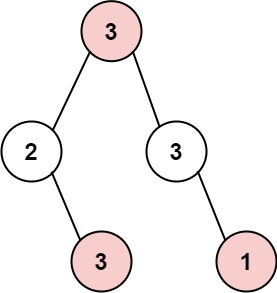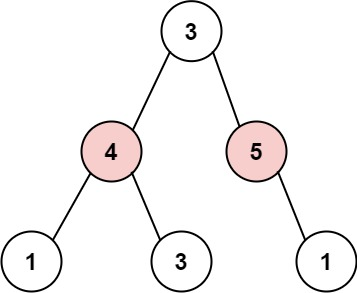304 North Cardinal St.
Dorchester Center, MA 02124

# House Robber III LeetCode Solution

## Problem – House Robber III LeetCode Solution

The thief has found himself a new place for his thievery again. There is only one entrance to this area, called `root`.

Besides the `root`, each house has one and only one parent house. After a tour, the smart thief realized that all houses in this place form a binary tree. It will automatically contact the police if two directly-linked houses were broken into on the same night.

Given the `root` of the binary tree, return the maximum amount of money the thief can rob without alerting the police.

Example 1:``````Input: root = [3,2,3,null,3,null,1]
Output: 7
Explanation: Maximum amount of money the thief can rob = 3 + 3 + 1 = 7.
``````

Example 2:``````Input: root = [3,4,5,1,3,null,1]
Output: 9
Explanation: Maximum amount of money the thief can rob = 4 + 5 = 9.
``````

Constraints:

• The number of nodes in the tree is in the range `[1, 104]`.
• `0 <= Node.val <= 104`

## House Robber III LeetCode Solution in C++

``````/**
* Definition for a binary tree node.
* struct TreeNode {
*     int val;
*     TreeNode *left;
*     TreeNode *right;
*     TreeNode(int x) : val(x), left(NULL), right(NULL) {}
* };
*/
class Solution {
public:
int tryRob(TreeNode* root, int& l, int& r) {
if (!root)
return 0;

int ll = 0, lr = 0, rl = 0, rr = 0;
l = tryRob(root->left, ll, lr);
r = tryRob(root->right, rl, rr);

return max(root->val + ll + lr + rl + rr, l + r);
}

int rob(TreeNode* root) {
int l, r;
return tryRob(root, l, r);
}
};``````

## House Robber III LeetCode Solution in Java

``````public class Solution {
public int rob(TreeNode root) {
int[] num = dfs(root);
return Math.max(num, num);
}
private int[] dfs(TreeNode x) {
if (x == null) return new int;
int[] left = dfs(x.left);
int[] right = dfs(x.right);
int[] res = new int;
res = left + right + x.val;
res = Math.max(left, left) + Math.max(right, right);
return res;
}
}
``````

## House Robber III LeetCode Solution in Python

``````class Solution(object):
def rob(self, root):
"""
:type root: TreeNode
:rtype: int
"""
def superrob(node):
# returns tuple of size two (now, later)
# now: max money earned if input node is robbed
# later: max money earned if input node is not robbed

# base case
if not node: return (0, 0)

# get values
left, right = superrob(node.left), superrob(node.right)

# rob now
now = node.val + left + right

# rob later
later = max(left) + max(right)

return (now, later)

return max(superrob(root))
``````
##### House Robber III LeetCode Solution Review:

In our experience, we suggest you solve this House Robber III LeetCode Solution and gain some new skills from Professionals completely free and we assure you will be worth it.

If you are stuck anywhere between any coding problem, just visit Queslers to get the House Robber III LeetCode Solution

Find on Leetcode

##### Conclusion:

I hope this House Robber III LeetCode Solution would be useful for you to learn something new from this problem. If it helped you then don’t forget to bookmark our site for more Coding Solutions.

This Problem is intended for audiences of all experiences who are interested in learning about Data Science in a business context; there are no prerequisites.

Keep Learning!

More Coding Solutions >>

LeetCode Solutions

Hacker Rank Solutions

CodeChef Solutions